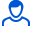Content: 00041.png (45.15 KB)

Positive responses: 0
Negative responses: 0

Sold: 0
Refunds: 0Loyalty discount! If the total amount of your purchases from the seller more than:

 \$1 the discount is 10% \$5 the discount is 30%

Determine the speed of wave propagation in an elastic medium if the phase difference of the oscillations of two points M and P, spaced from the source at distances y (M) = 20 m, and y (P) = 10 m, is equal to n / 2 radians. The oscillation phase of point M at time t1 = 0.1 s is equal to n radians. The initial phase of source oscillations is zero (source oscillations occur according to the sine law).
Task 00041. Detailed solution with a brief record of the conditions, formulas and laws used in the decision, the conclusion of the calculation formula and the answer.
If you have any questions about the decision, write. I will try to help. File in image format.
No feedback yet